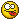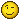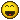mirror 3D transform?

Moderator: SecondMan

andromeda_girl
Fusionista
Posts: 320
Joined: Sun Feb 21, 2016 6:25 am
Been thanked: 1 time

mirror 3D transform?

what is the equivalent tool to a 2D transform in 3D when it comes to a flip?
I just want to do essentially the same thing as a 2D transform does when it comes to a horizontal flip / mirror but it seems the 3D FX tool does not have that as an option.thank you,
Au

SecondMan
Posts: 3346
Joined: Thu Jul 31, 2014 5:31 pm
Been thanked: 64 times
Contact:

Re: mirror 3D transform?

Negative scale?

Code: Select all

{
Tools = ordered() {
Shape3D3 = Shape3D {
Inputs = {
["Transform3DOp.Translate.X"] = Input { Value = -2.327, },
["Transform3DOp.Rotate.Z"] = Input { Value = 53.7, },
Shape = Input { Value = FuID { "SurfaceTorusInputs" }, },
["MtlStdInputs.MaterialID"] = Input { Value = 4, },
["SurfacePlaneInputs.ObjectID.ObjectID"] = Input { Value = 4, },
["SurfaceTorusInputs.ObjectID.ObjectID"] = Input { Value = 5, }
},
ViewInfo = OperatorInfo { Pos = { -1265, 412.5 } },
},
Mirror_X = Transform3D {
CtrlWZoom = false,
NameSet = true,
Inputs = {
SceneInput = Input {
SourceOp = "Shape3D3",
Source = "Output",
},
["Transform3DOp.ScaleLock"] = Input { Value = 0, },
["Transform3DOp.Scale.X"] = Input { Value = -1, },
},
ViewInfo = OperatorInfo { Pos = { -1155, 412.5 } },
},
Merge3D3 = Merge3D {
Inputs = {
SceneInput1 = Input {
SourceOp = "Shape3D3",
Source = "Output",
},
SceneInput2 = Input {
SourceOp = "Mirror_X",
Source = "Output",
},
},
ViewInfo = OperatorInfo { Pos = { -1155, 478.5 } },
}
}
}

andromeda_girl
Fusionista
Posts: 320
Joined: Sun Feb 21, 2016 6:25 am
Been thanked: 1 time

Re: mirror 3D transform?

unfortunately this did not solve my problem.
the mesh in question consists of two separate objects, so it was not possible to rescale them negatively in order to mirror them.
I ended up re exporting their mesh as individual objects and it fixed things.
maybe a 3D transform that has the same kind of mirror options like a 2D transform has might be sweetintelligent machine
Fusionista
Posts: 399
Joined: Fri May 13, 2016 10:01 pm
Location: Austin, Texas, USA
Been thanked: 28 times
Contact:

Re: mirror 3D transform?

It's not im-possible either:

Code: Select all

{
Tools = ordered() {
Shape3D3 = Shape3D {
Inputs = {
["SurfacePlaneInputs.ObjectID.ObjectID"] = Input { Value = 4, },
["Transform3DOp.Translate.X"] = Input { Value = -2.327, },
Shape = Input { Value = FuID { "SurfaceTorusInputs" }, },
["MtlStdInputs.Diffuse.Color.Green"] = Input { Value = 0, },
["MtlStdInputs.MaterialID"] = Input { Value = 4, },
["SurfaceTorusInputs.ObjectID.ObjectID"] = Input { Value = 5, }
},
ViewInfo = OperatorInfo { Pos = { -55, 16.5 } },
},
Shape3D3_1 = Shape3D {
Inputs = {
["SurfacePlaneInputs.ObjectID.ObjectID"] = Input { Value = 4, },
["Transform3DOp.Translate.X"] = Input { Value = -2.327, },
Shape = Input { Value = FuID { "SurfaceConeInputs" }, },
["MtlStdInputs.Diffuse.Color.Blue"] = Input { Value = 0, },
["MtlStdInputs.MaterialID"] = Input { Value = 4, },
["SurfaceTorusInputs.ObjectID.ObjectID"] = Input { Value = 5, },
["SurfaceConeInputs.ObjectID.ObjectID"] = Input { Value = 1, }
},
ViewInfo = OperatorInfo { Pos = { -55, 115.5 } },
},
Merge3D1 = Merge3D {
Inputs = {
SceneInput1 = Input {
SourceOp = "Shape3D3_1",
Source = "Output",
},
SceneInput2 = Input {
SourceOp = "Shape3D3",
Source = "Output",
},
},
ViewInfo = OperatorInfo { Pos = { 55, 82.5 } },
},
Transform3D1 = Transform3D {
Inputs = {
SceneInput = Input {
SourceOp = "Merge3D1",
Source = "Output",
},
["Transform3DOp.Rotate.X"] = Input { Value = 19.8, },
["Transform3DOp.Rotate.Y"] = Input { Value = -13.7, },
["Transform3DOp.Rotate.Z"] = Input { Value = 35.1, },
["Transform3DOp.PivotNest"] = Input { Value = 1, },
["Transform3DOp.Pivot.X"] = Input { Value = -2.327, },
["Transform3DOp.ScaleNest"] = Input { Value = 0, },
},
ViewInfo = OperatorInfo { Pos = { 165, 82.5 } },
},
Shape3D3_2 = Shape3D {
Inputs = {
["SurfacePlaneInputs.ObjectID.ObjectID"] = Input { Value = 4, },
["Transform3DOp.Translate.X"] = Input { Value = -2.327, },
["Transform3DOp.Rotate.X"] = Input { Value = 19.8, },
["Transform3DOp.Rotate.Y"] = Input { Value = -13.7, },
["Transform3DOp.Rotate.Z"] = Input { Value = 35.1, },
Shape = Input { Value = FuID { "SurfaceTorusInputs" }, },
["MtlStdInputs.Diffuse.Color.Green"] = Input { Value = 0, },
["MtlStdInputs.MaterialID"] = Input { Value = 4, },
["SurfaceTorusInputs.ObjectID.ObjectID"] = Input { Value = 5, }
},
ViewInfo = OperatorInfo { Pos = { -55, 280.5 } },
},
Mirror_X = Transform3D {
CtrlWZoom = false,
NameSet = true,
Inputs = {
SceneInput = Input {
SourceOp = "Transform3D1",
Source = "Output",
},
["Transform3DOp.ScaleLock"] = Input { Value = 0, },
["Transform3DOp.Scale.X"] = Input { Value = -1, },
},
ViewInfo = OperatorInfo { Pos = { 495, 16.5 } },
},
Merge3D3 = Merge3D {
Inputs = {
SceneInput1 = Input {
SourceOp = "Transform3D1",
Source = "Output",
},
SceneInput2 = Input {
SourceOp = "Mirror_X",
Source = "Output",
},
},
ViewInfo = OperatorInfo { Pos = { 495, 82.5 } },
},
Mirror_X_1 = Transform3D {
NameSet = true,
Inputs = {
SceneInput = Input {
SourceOp = "Merge3D1_1",
Source = "Output",
},
["Transform3DOp.ScaleLock"] = Input { Value = 0, },
["Transform3DOp.Scale.X"] = Input { Value = -1, },
},
ViewInfo = OperatorInfo { Pos = { 495, 313.5 } },
},
Merge3D1_1 = Merge3D {
Inputs = {
SceneInput1 = Input {
SourceOp = "Shape3D3_2",
Source = "Output",
},
SceneInput2 = Input {
SourceOp = "Shape3D3_3",
Source = "Output",
},
},
ViewInfo = OperatorInfo { Pos = { 165, 346.5 } },
},
Merge3D3_1 = Merge3D {
Inputs = {
SceneInput1 = Input {
SourceOp = "Merge3D1_1",
Source = "Output",
},
SceneInput2 = Input {
SourceOp = "Mirror_X_1",
Source = "Output",
},
},
ViewInfo = OperatorInfo { Pos = { 495, 379.5 } },
},
Shape3D3_3 = Shape3D {
Inputs = {
["SurfacePlaneInputs.ObjectID.ObjectID"] = Input { Value = 4, },
["SurfaceTorusInputs.ObjectID.ObjectID"] = Input { Value = 5, },
["Transform3DOp.Translate.X"] = Input { Value = -2.327, },
["Transform3DOp.Rotate.X"] = Input { Value = 19.8, },
["Transform3DOp.Rotate.Y"] = Input { Value = -13.7, },
["Transform3DOp.Rotate.Z"] = Input { Value = 35.1, },
Shape = Input { Value = FuID { "SurfaceConeInputs" }, },
["MtlStdInputs.Diffuse.Color.Blue"] = Input { Value = 0, },
["MtlStdInputs.MaterialID"] = Input { Value = 4, },
["SurfaceConeInputs.ObjectID.ObjectID"] = Input { Value = 1, }
},
ViewInfo = OperatorInfo { Pos = { -55, 379.5 } },
}
}
}


FBXMeshesMirror.zip
You do not have the required permissions to view the files attached to this post.

SecondMan
Posts: 3346
Joined: Thu Jul 31, 2014 5:31 pm
Been thanked: 64 times
Contact:

Re: mirror 3D transform?

andromeda_girl wrote:
Sun Feb 24, 2019 8:54 am
maybe a 3D transform that has the same kind of mirror options like a 2D transform has might be sweet
Sorry for taking so long to respond - I'm not quite getting what you are saying. What options are you talking about that are different than negative scaling?

andromeda_girl
Fusionista
Posts: 320
Joined: Sun Feb 21, 2016 6:25 am
Been thanked: 1 time

Re: mirror 3D transform?

I would have to send you the mesh as an example. depending on your mesh, your solution may or may not work.
but I was wondering about something -exactly- like what a 2d transform does. that would do it 100% of the time.

SecondMan
Posts: 3346
Joined: Thu Jul 31, 2014 5:31 pm
Been thanked: 64 times
Contact:

Re: mirror 3D transform?

Do -what exactly- 100% of the time?From your description so far, Flip Horizontal would be scale -1 in X, Flip Vertical would be scale -1 in Y.

Feel free to send a mesh where it doesn't work, and if possible an example of the result you are after.

Midgardsormr
Fusionista
Posts: 996
Joined: Wed Nov 26, 2014 8:04 pm
Location: Los Angeles, CA, USA
Been thanked: 46 times
Contact:

Re: mirror 3D transform?

You do need to center the mesh in the world first, then move it back afterward if you want to flip it in place rather than reflecting it across the origin.

SecondMan
Posts: 3346
Joined: Thu Jul 31, 2014 5:31 pm
Been thanked: 64 times
Contact:

Re: mirror 3D transform?

You don't need to - 3Xf has a pivot control:

Code: Select all

{
Tools = ordered() {
Transform3D1 = Transform3D {
CtrlWZoom = false,
Inputs = {
SceneInput = Input {
SourceOp = "Shape3D1",
Source = "Output",
},
["Transform3DOp.PivotNest"] = Input { Value = 1, },
["Transform3DOp.Pivot.X"] = Input { Value = -3, },
["Transform3DOp.ScaleLock"] = Input { Value = 0, },
["Transform3DOp.Scale.X"] = Input { Value = -1, },
},
ViewInfo = OperatorInfo { Pos = { 649, 19 } },
},
Shape3D1 = Shape3D {
Inputs = {
["Transform3DOp.Translate.X"] = Input { Value = -3, },
["Transform3DOp.Rotate.Z"] = Input { Value = -57.1, },
Shape = Input { Value = FuID { "SurfaceTorusInputs" }, },
["MtlStdInputs.MaterialID"] = Input { Value = 1, },
["SurfacePlaneInputs.ObjectID.ObjectID"] = Input { Value = 1, },
["SurfaceTorusInputs.ObjectID.ObjectID"] = Input { Value = 2, }
},
ViewInfo = OperatorInfo { Pos = { 539, 19 } },
}
}
}

SirEdric
Fusionator
Posts: 1723
Joined: Tue Aug 05, 2014 10:04 am
Real name: Eric Westphal
Been thanked: 77 times
Contact:

Re: mirror 3D transform?

When it comes to 'simple' ground reflections I normally just scale the Camera by Y-1.

Midgardsormr
Fusionista
Posts: 996
Joined: Wed Nov 26, 2014 8:04 pm
I've gotten so used to hacking things in a CustomVertex tool that I'd forgotten that!